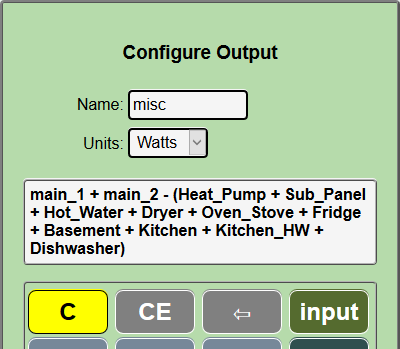# Outputs¶

Outputs provide useful values that are computed from input channel values using a calculator like interface. For instance, in a typical US installation, there are two MAIN circuits, the sum of the two is the total power into a panel. Its nice to know at a glance what that total is, but the two mains are measured separately using two input channels. We need a way to add them together to display the total usage.

Hover over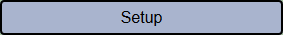and click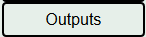in the dropdown buttons.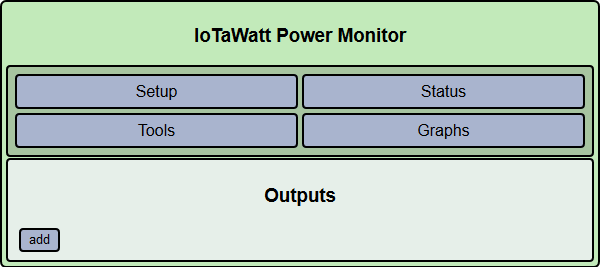This screen will list any outputs that you have already configured, and allow you to click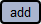to create new ones. You can click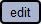on existing outputs to change or delete them. There is no practical limit to the number of outputs that you may create. The only requirement is that they be uniquely named.

So lets click: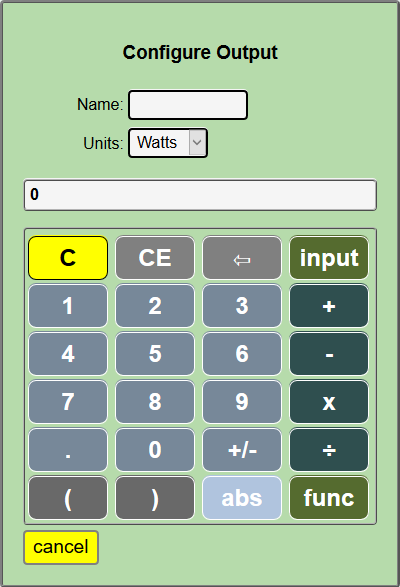This is the calculator interface that IoTaWatt uses to specify how to calculate an output using input channel values. A script is created that IoTaWatt uses to compute the value when needed. It works just like the simple four function calculators we are all used to, and using the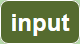key, you can select input channel values to be used in the formula that you are creating. The resulting expression is evaluated left to right, there is no operator hierarchy, but expressions within parenthesis will be evaluated first:

```1 + 2 x 3 will yield 9
1 + ( 2 x 3 ) will yield 7
```

Two additional functions min and max can be selected using the function button.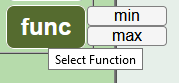These are binary functions just like + and x that operate on the operands to their left and right. The min function will return the lesser of the two operands and max the greater.:

```3 max 25 will yield 25
-4 min 0 will yield -4
-4 max 0 will yield 0
```

So lets make an output channel that combines two main inputs called main_1 and main_2. We enter the name total_power in the Name: box and hover over thebutton of the calculator to see a list of the inputs.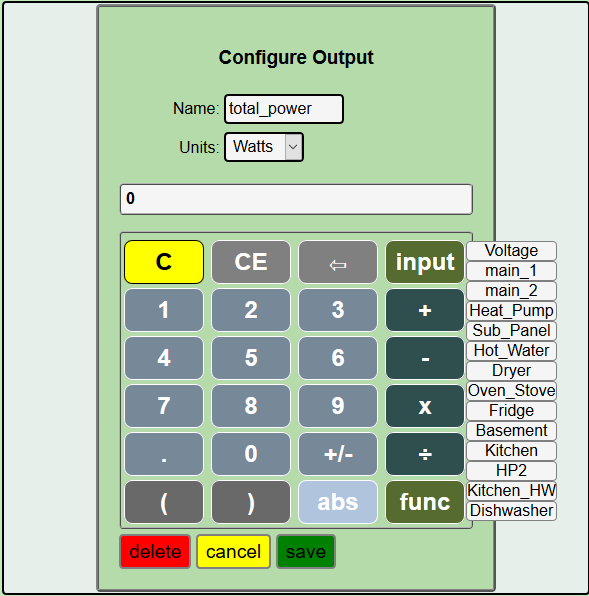Select main_1 from the list and it will appear in the calculator formula display. Next click on the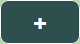, then repeat the input process selecting Main_2.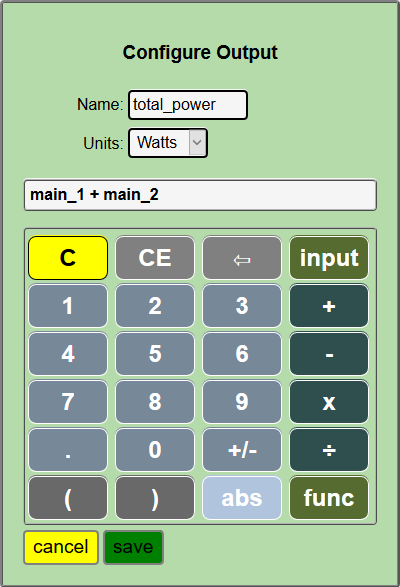Easy as that. Now press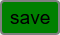to return to the outputs list. Your new output should appear within a second or two.Now go back to the Channels Status screen and see that the new output channel is listed and indeed has a value that is the sum of the two inputs main_1 and main_2.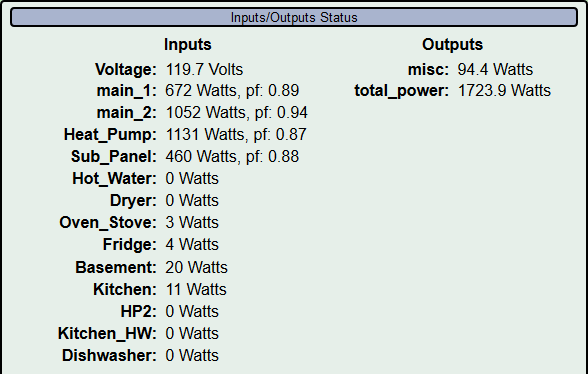Some other useful outputs would be:

• Power used in a solar PV system, calculated by adding the Solar inverter input to the (signed) Main input. If for instance the inverter were putting out 4500 watts and your Main(s) indicated an outflow represented as -3100 watts, local usage would be 1400 watts with 3100 watts exported.

• Where the Main(s) are monitored and selected circuits within the panel are also measured, you can create an output that shows the aggregate unmeasured usage by subtracting the measured inputs from the Mains as the misc output in the status display above. That output is defined: